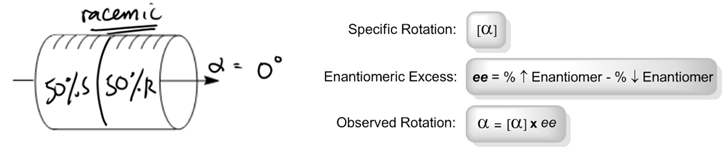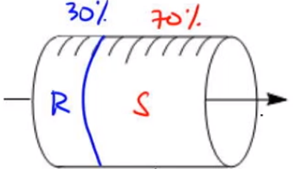## Organic Chemistry

Learn the toughest concepts covered in Organic Chemistry with step-by-step video tutorials and practice problems by world-class tutors.

5. Chirality

# Enantiomeric Excess

Some more facts about optical activity:

• Specific rotation [α] is the rotation that 100% pure enantiomers produce.
• Opposite enantiomer = opposite rotation.
• Racemic:A perfect 1:1 ratio of enantiomers
• Scalemic:A non-1:1 ratio of enantiomers
1
concept

## How to calculate enantiomeric excess.4m
Play a video:2
example

## Calculating Enantiomeric Excess and Observed Rotation50s
Play a video:
So my equation says that my observed rotation equals my specific rotation times my e. Okay, well, in this case, what was my specific rotation equal to? It was equal to positive. 20. Okay, so I just have to figure out what was e equal to e is equal to my highest and answer minus my lowest in anti mark. In this case, it was 50 minus 50 which meant that I had a Nancy America excess of zero. So that means that my e equals zero. So it was 20 times zero. Which means that even though I have this awesome specific rotation of 20 all of the Ananta MERS air getting canceled out, so there's no overall rotation at the end. So that is why my observed rotation zero.

Calculate the observed rotation when given the following ratio of enantiomers in solution.3
example

## Calculating Enantiomeric Excess and Observed Rotation2m
Play a video:
All right. So I'm gonna go ahead and take myself out of this videos that you guys can see the full image. So first of all, we had to figure out the Indians America access. The way we figure that out is by saying 70 minus 30% equals What percentage? 40% e. Okay, so that means that my e e is 40%. So what's my equation when my equation says that my observed rotation should be equal to my specific rotation, which is still positive 20 times my e, which is now gonna be 40%. Okay, Now, we don't really wanna work with percentages if we're multiplying. So we would turn this into 0.4, which is just I'm turning my my percentage into a decimal, which is very common. Okay, So now I would just multiply these together, and what I'm going to get is that 20 times 200.4 is gonna equal positive eight. Okay, so my Nancy America excess here should have been 40% and my observed rotation, instead of being positive 20 which is what it would be if it was 100. It's gonna be positive eight. Now, how does that intuitively make sense? Well, actually, it makes a lot of sense if you think about it. Remember what I said about Rosie mc Richy mc isn't optically active. Well, if I were to look at my tube as broken up into parts, what I would see is that the 70% is actually broken up into two parts. There's 30%. Here s that is perfectly canceled out by. 30% are. So what that means is that this entire area right here is Rosie. Mick, Okay. And the race Emmick portion adds up to I'm just gonna put here, Rossi, Mick, The race emmick portion adds upto what it adds up to 60% because I have 30 and 30. That means that the Onley part of this that's optically active. Let's put that back. That means that the only part of this that's optically active is the extra remaining s or the an anti American excess, and that part equals 40%. Okay, So that means that even though my s would love to rotate this positive 20 there's only 40% of it available that can actually rotate it because the other 30% is being canceled out by 30% of our Okay, So what that means is that Onley 40% is functional, and that's why it's only 40% of the total rotation is pop. That's happening out of the total rotation that I could have only 40% of that is actually taking place, so it's positive eight.

We can also use these equations to calculate specific and observed rotation when other variables are given to us. Just plug the numbers in! Let's try a few.

4
Problem

Calculate the observed rotation of a chiral mixture that contains 65% (S)-stereoisomer where the [α] of pure (S)-stereoisomer = -118.